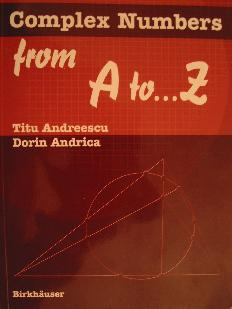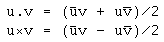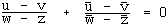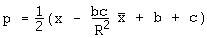Complex Numbers from A to ... Z by T. Andreescu and D. AndricaThe book reaches to about the same audience of (I quote from the back cover) "undergraduates, high school students and their teachers, mathematical contestants ... and their coaches, as well as anyone interested in essential mathematics," as some other books on the market, notably L.-s. Hahn's Complex Numbers & Geometry from the MAA, with which it has a nonempty overlap, naturally.

It's certainly not a book on Complex Analysis. As the authors mention in the Introduction, the symbol eit appears nowhere in the book. The book also misses on Möbius transform which takes about 20% of Hahn's book. It does not refer to any plane curves, not even cycloids. Still, I do not quite understand why the authors seem to have hesitated in adopting a less ambiguous title, say, "Complex Numbers from A to Z." The book is a real treasure trove of nontrivial elementary key concepts and applications of complex numbers developed in a systematic manner with a focus on problem solving techniques. Much of the book goes to geometric applications, of course, but there are also sections on polynomial equations, trigonometry, combinatorics ...

The book is organized into six chapters, Glossary, authors' and subject indices and a bibliography list. The chapters are as follows

1. Complex Numbers in Algebraic Form (pp. 1-28).
2. Complex Numbers in Trigonometric Form (pp. 29-52).
3. Complex Numbers and Geometry (pp. 53-88).
4. More on Complex Numbers and Geometry (pp. 89-160).
5. Olympiad-Caliber Problems (pp. 161-252).
6. Answers, Hints and Solutions to Proposed Problems (pp. 253-306).

Chapter 5's caption is somewhat misleading. It may create an impression that the previous chapters dealt with trivial matters or that Chapter 5 offers exclusively mathematical chestnuts only worthy of olympiad experience. Neither will be true. Problems constitute an integral part of the book alongside theorems, lemmas and examples. The problems are embedded in the text throughout the book, partly as illustrations to the discussed concepts, partly as the testing grounds for the techniques just studied, but mostly I believe to emphasize the centrality of problem solving in the authors' world view. Some problems, especially in chapters 3 and 4, have already been plucked from various olympiads and competitions: IMO, nationals, Putnam's ... . By the time the interested reader reaches Chapter 5, the time may be ripe to tackle "Olympiad-Caliber" problems. On the other hand, even Chapter 5 contains plenty of problems introductory in nature.

The book is really about solving problems and developing tools that exploit properties of complex numbers. The accumulation of the toolchest can be surmised from the subsection captions. For example Section 3.5 is split into: Equation of a line, Equation of a line determined by two points, The area of a triangle, Equation of a line determined by a point and a direction, The foot of a perpendicular from a point to a line, Distance from a point to a line. Section 4.6 sports such a sequence of subsections as The Distance OI, The Distance ON, The Distance OH. Aided by a subject index and a glossary, such a detailed table of contents makes the book very searchable. If you are looking for the formula for the area of a triangle, for example, then it is right there, in the table of contents. Characteristically, though, you'll find not only the formula itself, but also some of its applications. Say, the Area of a triangle subsection contains two solved problems of which one applies immediately to proving Menelaus' theorem.

In Chapter 4 the reader is treated to an unusual notions of the real and complex products of two complex numbers:These are none other but the scalar and vector products expressed in complex terms. E.g., u.u = |u|2. In a book on Complex Numbers their use is a real eye opener. I am aware of only one other book where complex numbers are mixed with the scalar product. This is Euclidean Geometry and Transformations by C. W. Dodge, where the basic object is the vector enhanced with the complex number symbolism. The book under review introduces the two products directly for the complex number objects. However simple and natural this usage is, this is something I have not seen done before. For example, the usual condition for the orthogonality of two lines UV and WZ (where a low case letter denotes the complex number corresponding to the point assigned the same letter in upper case) is commonly expressed asmeaning that in order for UV and WZ to be orthogonal the quotient (u - v)/(w - z) must be purely imaginary. (This is demonstrated in Chapter 3.) The common condition is immediately seen to be equivalent to (u - v).(w - z) = 0 which is more suitable for algebraic manipulation. It is also delightfully efficient. An application proved to be effortless. Say, two triangles are called orthologic if the perpendiculars from the vertices of one onto the "opposite" sides of the other are concurrent. If we deal with triangles ABC and A'B'C', then the orthogonality condition is given by

 (1) (z - a).(b' - c') = 0 (z - b).(c' - a') = 0 (z - c).(a' - b') = 0,

where z is just a variable. Taking it to be the point of concurrency, we add the three equations, eliminate z and obtain a concurrency condition

 (2) a.(b' - c') + b.(c' - a') + c.(a' - b') = 0.

This is because, going backwards, (2) is equivalent to

 (3) (z0 - a).(b' - c') + (z0 - b).(c' - a') + (z0 - c).(a' - b') = 0,

for any z0. Assuming z0 satisfies the first two equations in (1), we see from (3) that it also satisfies the third equation.

With little effort, (2) can be rearranged into

 (2') a'.(b - c) + b'.(c - a) + c'.(a - b) = 0

which shows that the relation is indeed symmetric. This fact is known as Maxwell's theorem after J. C. Maxwell who published a paper on the subject with an ingeniously beautiful proof (See, D. Pedoe Geometry: A Comprehensive Course, 114-116.) The theorem has also elicited interest from J. Steiner who proved it in 1827.

The reader will find a good deal of elegant and simple sample problems and even a greater quantity of technically taxing ones. The book supplies many great tools to help solve those problems. As the techniques go, the book is truly "From A to Z". Problem and example selection of course has been governed by authors' taste and experience. I would certainly include a lovely problem due to the late M. Klamkin (Mathematics Magazine, 28, 1955, 293)

 Prove that cos(5°) + cos(77°) + cos(149°) + cos(221°) + cos(293°) = 0.

Its omission is hardly a point of contention, though. But there is a couple of things worth complaining about. Annoyingly William Wallace's name is consistently misspelled as Wallance. It is misspelled in a short biographical footnote, in the Index and throughout the book whenever the Simson-Wallace line has been mentioned.

The formulation of Proposition 1 in section 4.5 is obviously incorrect:

Consider the point X(x) in the plane of triangle ABC (with the circumcenter at the origin.) Let P be the projection of X onto BC. Then the coordinate of P is given bywhere R is the circumradius of triangle ABC.

Clearly the projection of a point on BC does not depend on A, whereas R does. To correct the formulation, X is to be taken on the circumcircle of triangle ABC. The mishap, if noticed by the clever mathematical contestants, will probably amuse them more than disorient. On every occasion where the proposition is referred to, X is assumed to be in the right place. Less committed readers may get frustrated.

But the book is for a committed reader. It is for the readers who seek to harness new techniques and to polish their mastery of the old ones. It is for somebody who made it their business to be solving problems on a regular basis. These readers will appreciate the scope of the methodological detail the authors of the book bring to their attention, they will appreciate the power of the methods and the intricacy of the problems. Those who look for the "neglected mathematical beauty" may enjoy more the "guided tour" which is offered by L.-s. Hahn's book. A high school mathematics teacher and a team coach will do well owning both books, irrespective of their topical overlap.Complex Numbers from A to ... Z, by T. Andreescu and D. Andrica. Birkhäuser (2006), 321 pages, \$49.95. ISBN 0-8176-4326-5.